# firls

Least-squares linear-phase FIR filter design

## Syntax

b = firls(n,f,a) b = firls(n,f,a,w) b = firls(n,f,a,'ftype') b = firls(n,f,a,w,'ftype') 

## Description

firls designs a linear-phase FIR filter that minimizes the weighted, integrated squared error between an ideal piecewise linear function and the magnitude response of the filter over a set of desired frequency bands.

b = firls(n,f,a) returns row vector b containing the n+1 coefficients of the order n FIR filter whose frequency-amplitude characteristics approximately match those given by vectors f and a. The output filter coefficients, or “taps,” in b obey the symmetry relation.

These are type I (n odd) and type II (n even) linear-phase filters. Vectors f and a specify the frequency-amplitude characteristics of the filter:

• f is a vector of pairs of frequency points, specified in the range between 0 and 1, where 1 corresponds to the Nyquist frequency. The frequencies must be in increasing order. Duplicate frequency points are allowed and, in fact, can be used to design a filter exactly the same as those returned by the fir1 and fir2 functions with a rectangular (rectwin) window.

• a is a vector containing the desired amplitude at the points specified in f.

The desired amplitude function at frequencies between pairs of points (f(k), f(k+1)) for k odd is the line segment connecting the points (f(k), a(k)) and (f(k+1), a(k+1)).

The desired amplitude function at frequencies between pairs of points (f(k), f(k+1)) for k even is unspecified. These are transition or “don’t care” regions.

• f and a are the same length. This length must be an even number.

firls always uses an even filter order for configurations with a passband at the Nyquist frequency. This is because for odd orders, the frequency response at the Nyquist frequency is necessarily 0. If you specify an odd-valued n, firls increments it by 1.

The figure below illustrates the relationship between the f and a vectors in defining a desired amplitude response.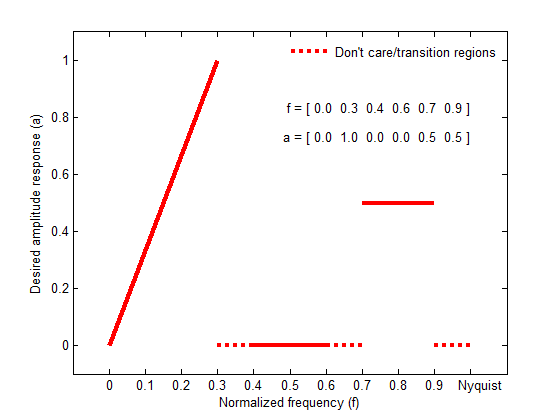b = firls(n,f,a,w) uses the weights in vector w to weight the fit in each frequency band. The length of w is half the length of f and a, so there is exactly one weight per band.

b = firls(n,f,a,'ftype') and

b = firls(n,f,a,w,'ftype') specify a filter type, where 'ftype' is:

• 'hilbert' for linear-phase filters with odd symmetry (type III and type IV). The output coefficients in b obey the relation

$b\left(k\right)=-b\left(n+2-k\right),\text{ }k=1,\dots ,n+1.$

This class of filters includes the Hilbert transformer, which has a desired amplitude of 1 across the entire band.

• 'differentiator' for type III and type IV filters, using a special weighting technique. For nonzero amplitude bands, the integrated squared error has a weight of (1/f)2 so that the error at low frequencies is much smaller than at high frequencies. For FIR differentiators, which have an amplitude characteristic proportional to frequency, the filters minimize the relative integrated squared error (the integral of the square of the ratio of the error to the desired amplitude).

## Examples

collapse all

Design an FIR lowpass filter of order 255 with transition band between $0.25\pi$ and $0.3\pi$. Use fvtool to display the magnitude and phase responses of the filter.

b = firls(255,[0 0.25 0.3 1],[1 1 0 0]); fvtool(b,1,'OverlayedAnalysis','phase')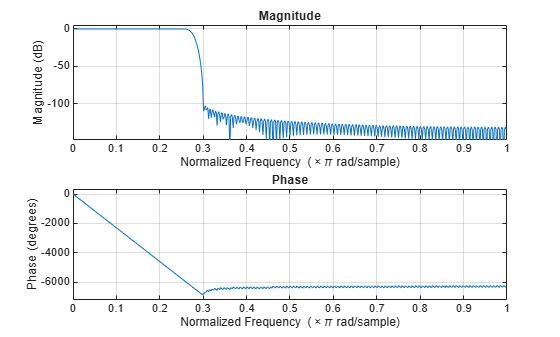An ideal differentiator has a frequency response given by $D\left(\omega \right)=j\omega$. Design a differentiator of order 30 that attenuates frequencies above $0.9\pi$. Include a factor of $\pi$ in the amplitude because the frequencies are normalized by $\pi$. Display the zero-phase response of the filter.

b = firls(30,[0 0.9],[0 0.9*pi],'differentiator'); fvtool(b,1,'MagnitudeDisplay','zero-phase')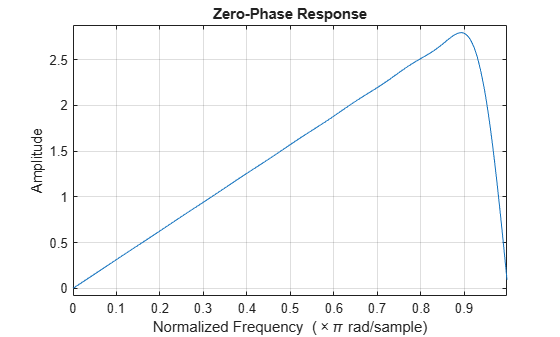Design a 24th-order antisymmetric filter with piecewise linear passbands.

F = [0 0.3 0.4 0.6 0.7 0.9]; A = [0 1.0 0.0 0.0 0.5 0.5]; b = firls(24,F,A,'hilbert');

Plot the desired and actual frequency responses.

[H,f] = freqz(b,1,512,2); plot(f,abs(H)) hold on for i = 1:2:6, plot([F(i) F(i+1)],[A(i) A(i+1)],'r--') end legend('firls design','Ideal') grid on xlabel('Normalized Frequency (\times\pi rad/sample)') ylabel('Magnitude')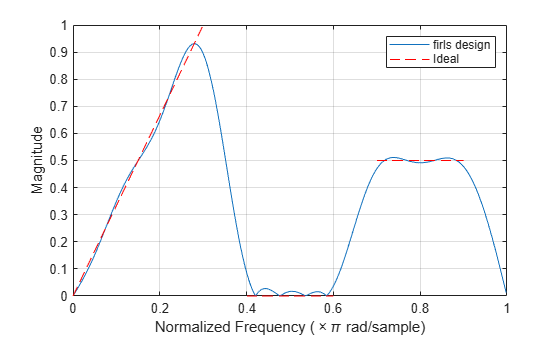Design an FIR lowpass filter. The passband ranges from DC to $0.45\pi$ rad/sample. The stopband ranges from $0.55\pi$ rad/sample to the Nyquist frequency. Produce three different designs, changing the weights of the bands in the least-squares fit.

In the first design, make the stopband weight higher than the passband weight by a factor of 100. Use this specification when it is critical that the magnitude response in the stopband is flat and close to 0. The passband ripple is about 100 times higher than the stopband ripple.

bhi = firls(18,[0 0.45 0.55 1],[1 1 0 0],[1 100]);

In the second design, reverse the weights so that the passband weight is 100 times the stopband weight. Use this specification when it is critical that the magnitude response in the passband is flat and close to 1. The stopband ripple is about 100 times higher than the passband ripple.

blo = firls(18,[0 0.45 0.55 1],[1 1 0 0],[100 1]);

In the third design, give the same weight to both bands. The result is a filter with similar ripple in the passband and the stopband.

b = firls(18,[0 0.45 0.55 1],[1 1 0 0],[1 1]);

Visualize the magnitude responses of the three filters.

hfvt = fvtool(bhi,1,blo,1,b,1,'MagnitudeDisplay','Zero-phase'); legend(hfvt,'bhi: w = [1 100]','blo: w = [100 1]','b: w = [1 1]')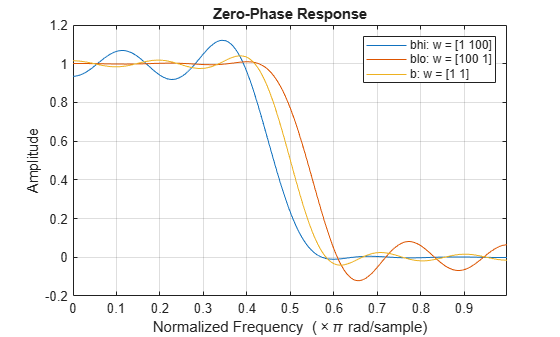## Diagnostics

One of the following diagnostic messages is displayed when an incorrect argument is used:

F must be even length. F and A must be equal lengths. Requires symmetry to be 'hilbert' or 'differentiator'. Requires one weight per band. Frequencies in F must be nondecreasing. Frequencies in F must be in range [0,1]. 

A more serious warning message is

Warning: Matrix is close to singular or badly scaled. 

This tends to happen when the product of the filter length and transition width grows large. In this case, the filter coefficients b might not represent the desired filter. You can check the filter by looking at its frequency response.

## Algorithms

Reference  describes the theoretical approach behind firls. The function solves a system of linear equations involving an inner product matrix of size roughly n/2 using the MATLAB® \ operator.

This function designs type I, II, III, and IV linear-phase filters. Type I and II are the defaults for n even and odd respectively, while the 'hilbert' and 'differentiator' flags produce type III (n even) and IV (n odd) filters. The various filter types have different symmetries and constraints on their frequency responses (see  for details).

Linear Phase Filter TypeFilter OrderSymmetry of CoefficientsResponse H(f), f = 0Response H(f), f = 1 (Nyquist)

Type I

Even

$b\left(k\right)=b\left(n+2-k\right),\text{ }k=1,...,n+1$

No restriction

No restriction

Type II

Odd

$b\left(k\right)=b\left(n+2-k\right),\text{ }k=1,...,n+1$

No restriction

H(1) = 0

Type III

Even

$b\left(k\right)=-b\left(n+2-k\right),\text{ }k=1,...,n+1$

H(0) = 0

H(1) = 0

Type IV

Odd

$b\left(k\right)=-b\left(n+2-k\right),\text{ }k=1,...,n+1$

H(0) = 0

No restriction

## References

 Parks, Thomas W., and C. Sidney Burrus. Digital Filter Design. New York: John Wiley & Sons, 1987, pp. 54–83.

 Oppenheim, Alan V., Ronald W. Schafer, and John R. Buck. Discrete-Time Signal Processing. Upper Saddle River, NJ: Prentice Hall, 1999.